# Quiz 15: Managing Service Inventory

Business

In the present case there is consulting firm that provides consultancy services to its client. The company is located at more than 300 locations in the United States as well as worldwide. The work requires that the employees of the company have to continuously interact with the clients and this result in developing of cordial relation between the employees and the clients. The employees could not be stopped from interacting with the clients as it is the part of the job and in order to make the clients satisfied there has to be cordial relation between the employees and the clients. The employees are also highly qualified and this results in offer of very good jobs from the clients to the employees. Thus, the attrition rate at the company is very high. The company employs a training program for the employees every year and recruits employees in the month of June so that the employees could attend the training program. The training expenses of the employees per year are \$850,000. The numbers of employees that are recruited each year are 180. Each person that is recruited earns \$90,000 per year. The attrition rate is 3.5 persons per week. Number of employees that should be recruited and enrolled in each session The number of employees that should be recruited and should be enrolled in a training session can be determined by using the economic order quantity model. The formula of the economic order quantity is given below: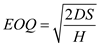…… (1) Here, D = Demand in units per year. H = Holding cost in dollars per unit per year. S = Cost of placing an order in dollars per order. Q = Order quantity in units. In the given case the demand for the employees is about 180 employees per year. The cost of placing the order is \$90,000.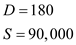Holding cost in dollars per unit per year can be calculated by dividing the cost of training the employees in a year by the number of employees that are recruited. Therefore, the holding cost calculated is shown below:Thus, the values can be put in equation (1) given above:Thus, around 83 people can be recruited at a time. It is given that the attrition rate is 3.5 persons per week. Thus, the number of weeks in which 83 people can be recruited can be calculated as given below:Thus, 83 people would be required at the end of 24 weeks, which means that there would be requirement of 83 people at the end of 6 months. Thus, in total 180 people can be recruited at two times in a year and two training sessions could be held for the people recruited.

In the given case there is a company that is a wholesale distributor of bicycles. The company distributes the bicycle through its eight primary retail outlets. The company has a wide variety of bicycles that are dependent on five different frame sizes. The table given below gives the list price of five different frames: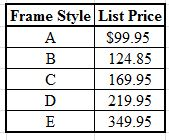Table 1.1 The company receives the different styles of bicycles from a single manufacturer. The cost of placing the order of bicycles with the manufacturer is \$65. The cost price per cycle is roughly around 60 % of the list price. The holding cost of the bicycles is around 0.75 % of cost price of bicycles per month. The table given below gives the monthly demand of bicycles of various frames.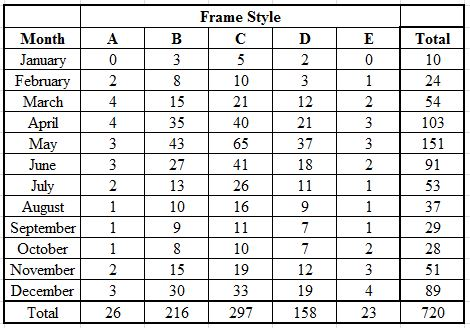Table 1.2 An Economic Order Quantity (EOQ) model is used in cases where the demand is constant and there are no stock outs. The EOQ model gives very accurate results related with the quantity that must be ordered. The ability to supply additional stocks to the retail outlet gives the company a competitive edge over its rivals. Thus, the company must maintain additional stock at its distribution center in order to cater to the demand and that there should be no stock out at the center. Thus, an economic order quantity model could be used to determine the exact stock levels for each month at the center. The formula of the economic order quantity is given below:…… (1) Here, D = Demand in units per year. H = Holding cost in dollars per unit per year. S = Cost of placing an order in dollars per order. Q = Order quantity in units. The demand of different types of frames for each month is given in table 1.2. The holding cost is 0.75% of the list price. The list price for each type of frame is given in table 1.1. The holding cost for each frame type can be calculated and is shown in the table given below: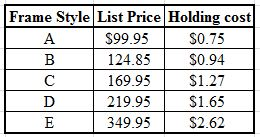Table 1.3 Thus, the table given above gives the holding cost of five different types of frames. The cost of placing an order is given out to be \$65. Thus, by using the formula as given in equation (1), the quantity that must be order in the month of January for the frame of type A can be calculated as given below:Thus, by using the same formula and the given quantities the quantity to be ordered could be calculated for the rest of the months and in similar manner the quantity to be ordered for other frame types and for the given months could be calculated. The table given below shows the quantity to be maintained for the frame types A, B and C.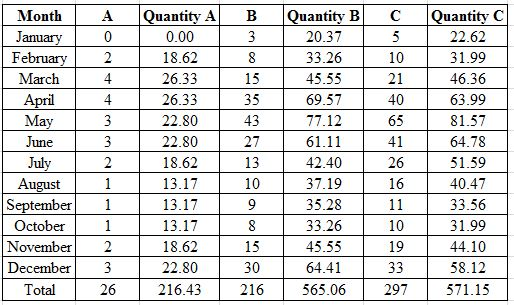Table 1.4 The formulas that are used in the excel sheet in order to calculate the values as obtained in the table given above are shown below: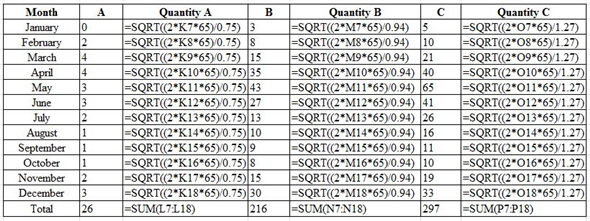Table 1.5 Similarly the quantities of frame type D and E that are required to be maintained at the distribution centers can be calculated and are shown in the table given below: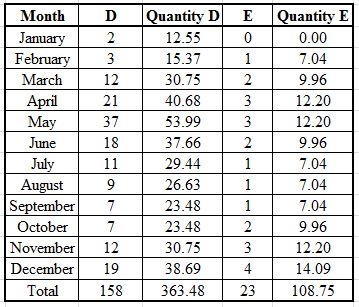Table 1.6 The formulas that are used in the excel sheet in order to calculate the values as obtained in the table given above are shown below: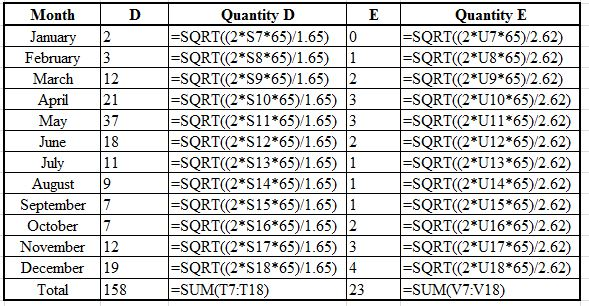Table 1.7 Thus, the quantities of frames A, B, C, D and E to be maintained at the distribution center can be summarized in the table given below: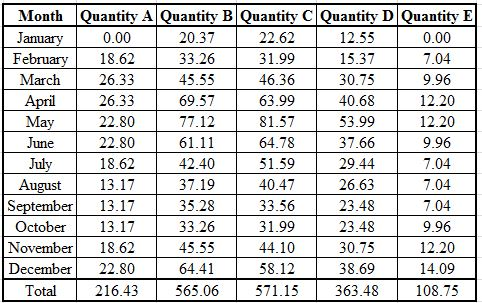Table 1.8 Thus, the table as shown above gives the quantities of frames A, B, C, D, and E that needs to be maintained at the distribution center. A service level of 95 percent is required to be maintained at the company. The lead time is 4 weeks. The standard deviation for each frame type could be calculated by calculating the variance for each frame type as shown in the table given below: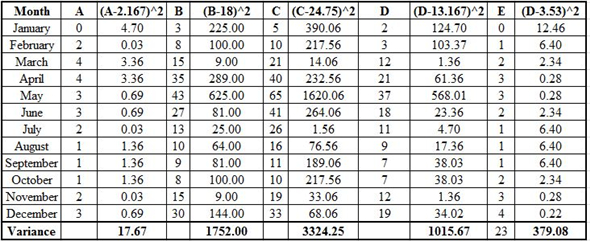Table 1.9 Thus, the variance for frame type A is 17.67, B is 1752, C is 3324.25, D is 1015.67 and E is 379.08. Thus, standard deviation for each frame type can be calculated by using the formula for standard deviation as shown below: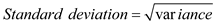…… (2) Therefore, standard deviation for frame A will be,Similarly the standard deviation of other frames types could also be calculated and are shown in the table given below: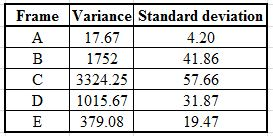Table 1.10 The formula used for calculating the safety stock is given below: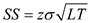…… (3) Here, SS = Safety stock. Z = Normal distribution value for a given service level.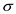= Standard deviation. LT = Lead time. Thus, for a service level of 95 percent the value of z is 1.645, the lead time is of 4 days and the values of standard deviation for various frame types are given in table 1.10. Thus, the value of safety stock can be calculated by using formula as given in equation (3). Safety stock for frame AThus, similarly, the safety stock for other frame types can also be calculated and are given in table as shown below: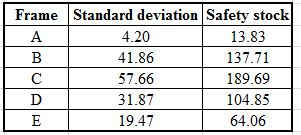Table 1.11 Thus, the safety stock calculated for various frames are shown in the table given above.

This question can be answered with the single-period model for perishable goods Equation (15).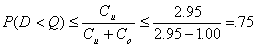where:This assumes that the loss incurred in the stockout of one dessert is the contribution.  The cost of overage is assumed to be the out-of-pocket cost to produce one additional dessert wasted if not sold.  Ordering the 20 observed weeknight demands in ascending order we develop the demand distribution below with cumulative probability P(D) shown in the second row (note that two values of 250 and 275 were observed).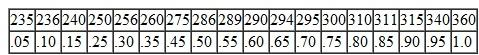Thus, stocking 300 deserts satisfies our critical fractile of 0.75.